## 8.08.2022

### Multiplication by zero and zero element

Mathematicians claim that when multiplied by zero, multiplication occurs and "multiplying by zero (zero element) gives a number equal to zero." Let's look at a couple of examples.

## Zero on the football field

We go to the stadium to watch European football. This is exactly what blondes need. Firstly, beautiful gerls are shown on TV. Secondly, in European football, twenty-two millionaires kick the same ball with varying degrees of success. Where else will blondes find so many rich suitors in one place? And so, the game is in full swing. One player has broken the rules and is removed from the field. What is left on the field instead of this player? An empty space that cannot take part in the game. If the players are numbers, then the empty space is zero. Empty space is not a player, zero is not a number.

Now consider the "null element", which is no different from the "numeric elements". The same football match, the same situation - the player was sent off. And here is the main trick - instead of a remote player, a "zero player" with the number "zero" on a T-shirt enters the field. He joins the game and soon scores a goal. This is where "higher mathematics" begins. One team proves that the zero player is exactly the same as the rest of the players, therefore, has the right to score goals. The other team proves that this is a removed player and he does not have the right to score goals. Here's a great excuse for you to start a "special military operation" like a "football war" between El Salvador and Honduras in 1969. The idiocy of such a situation needs no comment.

## Multiplication by zero in the store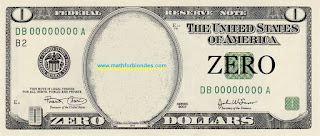Zero dollars

Another example from our life. We all go to the store and buy something. What is the buying process? This is the exchange of the money we have for the goods available in the store. The buying process itself can be compared to multiplication. If the buyer has money, and the store has goods, there are no problems. If the customer doesn't have the money or the store doesn't have the product, then you can't make the purchase. You wouldn’t go to the store with an empty wallet to hear from the seller that you can’t buy anything without money? This situation can be seen as an example of multiplication by zero.

Now consider the process of buying with "zero element". Imagine that you have a bill in your wallet that says "zero dollars". You go to the store with this bill and exchange it for a piece of paper with the inscription "zero goods". Technically, you made a purchase without having a cent in your pocket and without buying anything. Mathematicians tell us about a similar “multiplication by zero (zero element)”.

## Multiplication by zero is not possible

The substitution of concepts can change our logic beyond recognition. This modified logic forces us to look for multiplication by zero where it cannot be - in the results of multiplication. Since multiplication by zero does not occur, then you need to look not into the void (after all, there is no result of multiplication by zero), but into the initial conditions of multiplication. Two apples both lay and will remain lying, even after casting the spell "Apples, I multiply you by zero." Mathematically, this is written to the point of banality simply:

2*0=2*0

All this happens because our mathematicians have not learned to adequately describe reality with the help of mathematics. If you want to look at multiplication examples taken from real life, then you can do it here.

We will consider multiplication by zero in geometry and physics in more detail, to be continued.

P.S. What should you do? Remember that zero is not a number. And when it comes to zero in mathematics, discard your logic and common sense and open the Holy Mathematical Scripture. What is written about the case you are interested in, then tell the mathematicians. You will not begin to assert in a theological seminary that there is no God. So I do not recommend arguing with mathematicians - this is fraught with serious consequences for you. When you become adults and mathematicians disappear from your life, then you can say what you think is right.

## 7.28.2022

### Multiplication by zero

In the comments to the article "Multiplication by zero" of the Russian version of this site, I was asked an interesting question:

Nikolai, I read the article halfway, but still ... There are two apples in front of me (fact). Further, I, like a "sorcerer", multiply them by zero and still see two apples in front of me! Although, according to the laws of arithmetic, they should have disappeared from me! What does mathematics say about this? Thanks for the answer.Two apples

Here they are, beauties. They lie down and smile. Like, well, what do you say to that? So what is multiplication by zero? Let's try to figure this out.

Pay attention, the question is formulated very cleverly: not "what do mathematicians say?", but "what does mathematics say?". The first question is the easiest to answer. Preachers say, "Read the Bible," mathematicians say, "Read the Definition." They answer stupidly. Nobody needs to explain anything. And the meticulous can always, with a smart look, hang noodles on their ears.

Next, we will consider the situation from the position of the "sorcerer". The sorcerer declares that he will multiply the apples by zero. Then the sorcerer says: "Close your eyes and do not open." While your eyes are closed, the sorcerer hides the apples. "Open your eyes. See the apples? They are not there. The great miracle of multiplication by zero has happened - the apples have disappeared!" The sorcerer-mathematician will surely add: "What was required to be proved."

Now a few words about mathematicians. They, like proud eagles, soar high in the clouds of their abstract ideas. Mathematicians descend to our sinful earth only when they see food - a problem that they can solve. Mathematicians have learned very well to tear numbers from reality and perform various manipulations with them. When it becomes necessary to bring numbers back to reality, sometimes very big problems arise. Multiplying by zero is one such problem.

Let's start from the very beginning. The Russian-language Wikipedia page in one of the versions wrote: "Multiplication is one of the main binary mathematical operations (arithmetic operations) of two arguments (multiplicand and multiplier), the result of which is a new number (product). ... Multiplication by zero (zero element) gives a number equal to zero: x ⋅ 0 = 0".

If we translate the above quote into ordinary human language, then two elements (multiplicand and multiplier) are needed for multiplication. After multiplying them, a new element will be obtained, which is the result of multiplication. It is customary to write it like this:

a*b=c

On the left side of the equal sign is written what precedes the multiplication. The result of the multiplication is written on the right side. One element is multiplied by another element, resulting in a third element.

If we consider the logic of mathematicians, then calling zero the "zero element", all the "laws" of multiplication are observed - when multiplied by the zero element, all other elements turn into the zero element. There is only one question left: "Where do the apples go?".

Now I will present my own view on the problem of multiplication by zero. First read my reasoning, and at the end I will give practical recommendations on how to use my new knowledge. So what does the math say about multiplying by zero?

From the point of view of mathematics, multiplication by zero is impossible, since the multiplication itself does not occur. If in my earlier works I stated something else, then I was mistaken. The process of cognition is continuous and what seemed right to me yesterday may look completely different today.

The positional notation of numbers looks like this: units, tens, hundreds... If there is a number in the positional notation, then we write it down. For example, 324 is three hundred, two tens, four ones. And if there is no number in a separate position? What then? We write zero instead of the number that is missing. For example, 304 is three hundred, no tens, four units. I affirm that the absence of a number cannot be a number. In other words, zero is not a number and the rules of numbers do not apply to it.

In the multiplication example, zero represents an empty space in the place of one of the factors and an empty space in the result of the multiplication. Multiplication, as a mathematical operation, does not occur. It's like trying to clap with one hand. To get sound, there must be two palms. You see how smart we have become: we have determined that clapping is a binary operation that can be described by the mathematical operation of multiplication:

[one palm]*[other palm]=[applause]

1[palm]*1[palm]=1[applause]

Now let's remove one palm. In our mathematical expression, we will replace one of the palms with zero and look at the result.

0[palm]*1[palm]=0[applause]

1[palm]*0[palm]=0[applause]

In order for the multiplication to occur, we need two completely different palms, and not the same one. Mathematicians tell us that when a number is raised to the second power, it multiplies itself. It is just as impossible to multiply a number by itself as it is impossible to create an applause with one palm.

You can say that in audio equipment, one speaker can produce sound, the second speaker is not needed for this. In the case of a speaker, there is another multiplication formula: the speaker is multiplied by the electric current and the result of the multiplication is sound.

1[speaker]*1[electric current]=1[sound]

If there is no speaker (for example, it is broken), the electric current cannot produce sound.

0[speaker]*1[electric current]=0[sound]

If there is no electric current (Putin cut off the wires), the speaker cannot produce sound.

1[speaker]*0[electric current]=0[sound]

In ordinary human language, the operation of multiplication by zero can be translated as follows:

0*b=0

Multiplication does not occur because there is nothing to multiply, the result of multiplication is absent.

a*0=0

Multiplication does not occur because there is nothing to multiply by, the result of multiplication is absent.

Something like this. Next time I will tell you about the zero element in multiplication.

## 1.29.2022

### Squaring the circle

Squaring the circle. The solution of the problem. Almost accurate. Math draw.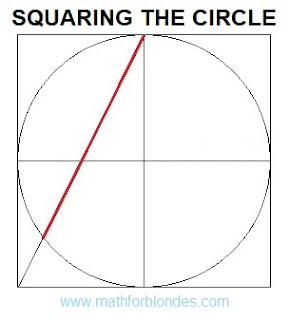Squaring the circle. The solution of the problem. Almost accurate.

This construction is not a solution to the problem of squaring the circle, but it forced me to get my hands on the math and do all the verification calculations. If the correct solution should give a segment with a length of 1.77245 radii, then the length of the red segment in the figure is 1.78885 radii. A little more than necessary. How much more? The segment exceeds the required length by 0.9253% or by 0.01640 of the radius. The area of a square with sides equal to the length of this segment is 1.8592% greater than the area of a circle.

How to use it? For a friendly prank of math lovers. Build a square with sides equal to ten centimeters. Inscribe in a square a circle with a radius of 5 centimeters. Draw a line as shown in the figure and offer a ruler to measure the length of the resulting segment. It will be equal to 8.9 centimeters. Using a calculator, calculate the area of a circle, which is equal to 78.54 square centimeters. The area of a square with sides of 8.9 centimeters is 79.21 square centimeters. This discrepancy can be explained by the low accuracy of measurements using a ruler. Personally, after such rough calculations, I sat down to check the solution.

I don't recommend this kind of joke with my teachers or math teachers - they can force you to do all the verification calculations to refute this solution. The unsolvability of this problem was proved by the German mathematician Lindemann back in 1882. However, I have long been very distrustful of many proofs of mathematicians.

## 1.01.2022

### Signs greater than and less than

How to remember signs greater than and less than? A very long time ago, when I was little and went to school, the teacher taught us this. Even as an adult, I have always used this method.

We always do more with our right hand than with our left. If we bend our right arm at the elbow and raise it above our head, we get the "GREATER THAN" sign. We do less with our left hand. A bent and raised left hand will give us the "LESS THAN" sign. The elbow of the right hand points to the direction of the "GREATER THAN" sign. This is how it looks in the picture.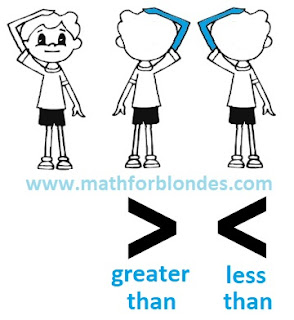Signs greater than and less than

Indeed, the arm bent at the elbow is very similar to the "greater than" and "less than" symbols. You don't even have to raise your hand. And this cheat sheet will always be with you. If mathematicians don't cut your hands off.

If you are left-handed and do more with your left hand than with your right? How then to be? You have two options. Or you think like everyone else and you won't have any problems with math. Or you write your "left-handed math" and learn a lot of interesting things.

## 10.30.2021

### 2 to the power zero five

How much is 2 to the power zero five? A trick question, like everything mathematicians come up with for us. In short, transformations look like this.2 to the power zero five

Now let's take a closer look at the logic of mathematicians. Raising a number to an integer power is the multiplication of these numbers. Raising a number to a fractional power is extracting the root of a number, with the number in the denominator showing which root to find. But the fraction itself can be written both in the form of a decimal fraction, and in the form of a regular fraction. We put all this in a pot, mix thoroughly and the mathematical puzzle for the textbook is ready.

You need to solve this puzzle in the following order. We convert the decimal fraction zero five into a regular fraction and get one second. Now we can write the number 2 with a fractional exponent as the square root of two and calculate its value.

To consolidate knowledge, consider two more simple examples.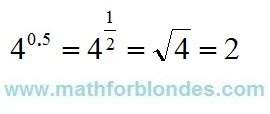4 to the power zero five

4 to the power zero five is the same as 4 to the one-second power. Four to the power of one second is the square root of 4. Taking the square root of four gives two.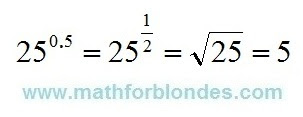25 to the power zero five

After considering the two previous examples, it will be very easy to calculate 25 to the power zero five. As real shamans, we turn zero five into one second, extract the square root of 25 and get the desired result - the root of twenty-five is five.

Why all these dances of shamans with tambourines? There are two sides to this math medal. Front side - mathematicians teach us to use mathematics. The other side of the coin is that if mathematicians simply and clearly express their thoughts in the language of mathematics, then they will not seem so smart to us, and we ourselves will not look such fools.

## 10.16.2021

How to find 101 cubed using abbreviated multiplication formulas? I have already shown how, using the cubes of the sum formula, one can calculate one hundred and one in a cube. There I presented one number 101 as the sum of two numbers 100 and 1. The use of simpler (more convenient in calculations) numbers makes the calculation easier.

On the Internet, I saw an example of using other formulas, the sum of cubes and the difference of cubes. This is a bad example. To find 101 cubed, use the numbers 101 and 100. This is how it looks.101 cubed. Bad example.

Why is this a bad example? I'll show you now. I'll take the number 101, the cube of which we need to find, and zero.101 cubed. Removing garbage.

By using zero, I removed all the mathematical garbage from the calculations - these are four multiplications and four additions or subtractions.

## 7.25.2021

### Cube of 101

Today we will look at an example of how you can find one hundred and one cubed using the abbreviated multiplication formulas. In other words, how to raise cube of 101 using the abbreviated multiplication formula.

The following cry for help was heard in the comments:

Help please, problem (example) from the physics and mathematics school (Russia), grade 8:

101^3

I don't understand how to solve this with abbreviated multiplication formulas.

It is very easy to cube the number 101 - you need to type the number 101 on a calculator and multiply twice by the same number 101.

101^3=101*101*101=1030301

If you don't have a calculator at hand (you never know, the phone has just been stolen), then you can calculate on a piece of paper in a column (the picture will be at the end, like checking both the calculator and the formula).

## Cube of 101 We apply the cube of sum formula.

The problem statement says that you need to find the cube of 101 using the abbreviated multiplication formula. According to math teachers, everyone should know these formulas. Naive. Where to find this formula?

You can search the Internet for a cube of sum. Google to help you. You can find these formulas in a reference book on mathematics, in a textbook on mathematics, you can ask a classmate who knows the formulas for abbreviated multiplication by heart. The formula for the abbreviated multiplication we need is called cube of sum. You will see it below.

And now the answer to the most tricky question: how to get the sum of numbers from one number? It is necessary to expand this number into terms. From the point of view of mathematics, the number of terms can be any, but ... I found the abbreviated multiplication formulas for raising a sum to a cube only for two and three terms. The formula for the cube of the sum of three terms is very complicated, I wish you never to face such a thing. But the cube of the sum of the two terms looks nice. The basic principle of expansion into terms for applying abbreviated multiplication formulas is that numbers can be easily multiplied in your head without using a calculator. For the number 101, the best option would be 101 = 100 + 1. 100 and 1 numbers is easy to multiply without a calculator. Let's see what we get.Cube of 101

I don't know about you, but I couldn't do without a piece of paper. Yes, I wrote everything down in a line, not a column, but nevertheless. And in conclusion, let's check our solution by multiplying in a column on a piece of paper.Cube of 101 in a column on a piece of paper

Well, something like this. Why do you need all this? So that you know that you can multiply not only on a calculator or in a column, but sometimes you can effectively use abbreviated multiplication formulas. If, of course, you know them.